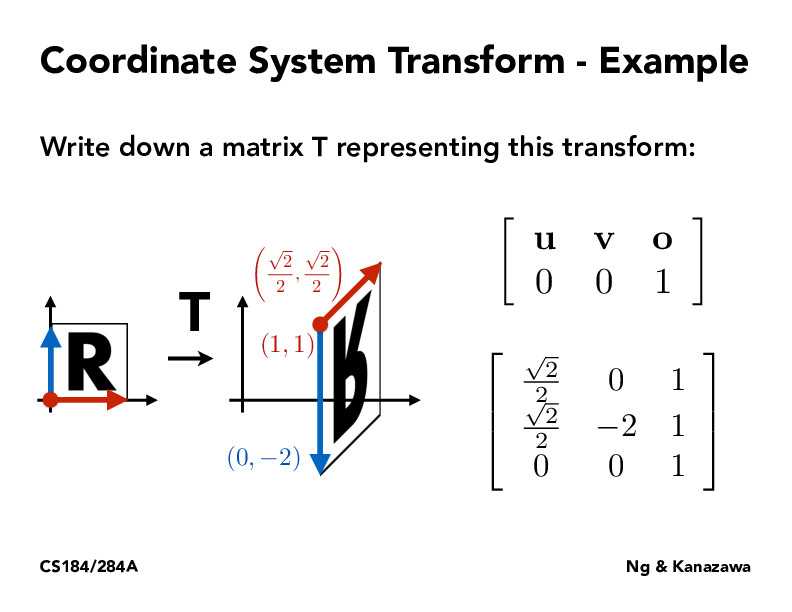Lecture 4: Transforms (41)SainanChen

In the beginning, I was confused about what the input vector and output vector represent for transform matrix $\mathbf{T} * (x,y,1)^T = (x',y',1)^T$. Then I realized if $\mathbf{u}$, $\mathbf{v}$ are 2 direction vectors and $\mathbf{o}$ is a point (based on original coord system), they form a new coordinate system. $(x,y)$ is coordinate of point based on the new coord system, and $(x',y')$ is coordinate of the point based on original coord system.yirenng

@SainanChen -- thanks for this helpful explanation!

Also, this website allows you to write comments in Markdown, which lets you include hyperlinks, typeset equations professionally using LaTeX syntax, and more. I've edited your comment above to use Markdown for the equations, and encourage you and other students to try Markdown for your comments going forward!

You must be enrolled in the course to comment